# Mask Compositing: The Crash Course

DataStax Astra — Open, multi-cloud stack for modern apps

At the start of 2018, as I was starting to go a bit deeper into CSS gradient masking in order to create interesting visuals one would think are impossible otherwise with just a single element and a tiny bit of CSS, I learned about a property that had previously been completely unknown to me: `mask-composite`.

As this is not a widely used property, I couldn’t find any comprehensive resources on this topic. So, as I began to use it more and learn more about it (some may remember I’ve mentioned it before in a couple of other articles), I decided to create such a resource myself and thus this article was born! Here, I’m covering how `mask-composite` works, why it’s useful, what values it can take, what each of them does, where we are in terms of support and what alternatives we have in non-supporting browsers.

### What mask compositing does

Mask compositing allows us to combine different `mask` layers into a single one using various operations. Combine them how? Well, pixel by pixel! Let’s consider two `mask` layers. We take each pair of corresponding pixels, apply a certain compositing operation (we’ll discuss each possible operation in detail a bit later) on their channels and get a third pixel for the resulting layer.How compositing two layers works at a pixel level.

When compositing two layers, the layer on top is called the source, while the layer underneath is called the destination, which doesn’t really make much sense to me because source sounds like an input and destination sounds like an output, but, in this case, they’re both inputs and the output is the layer we get as a result of the compositing operation.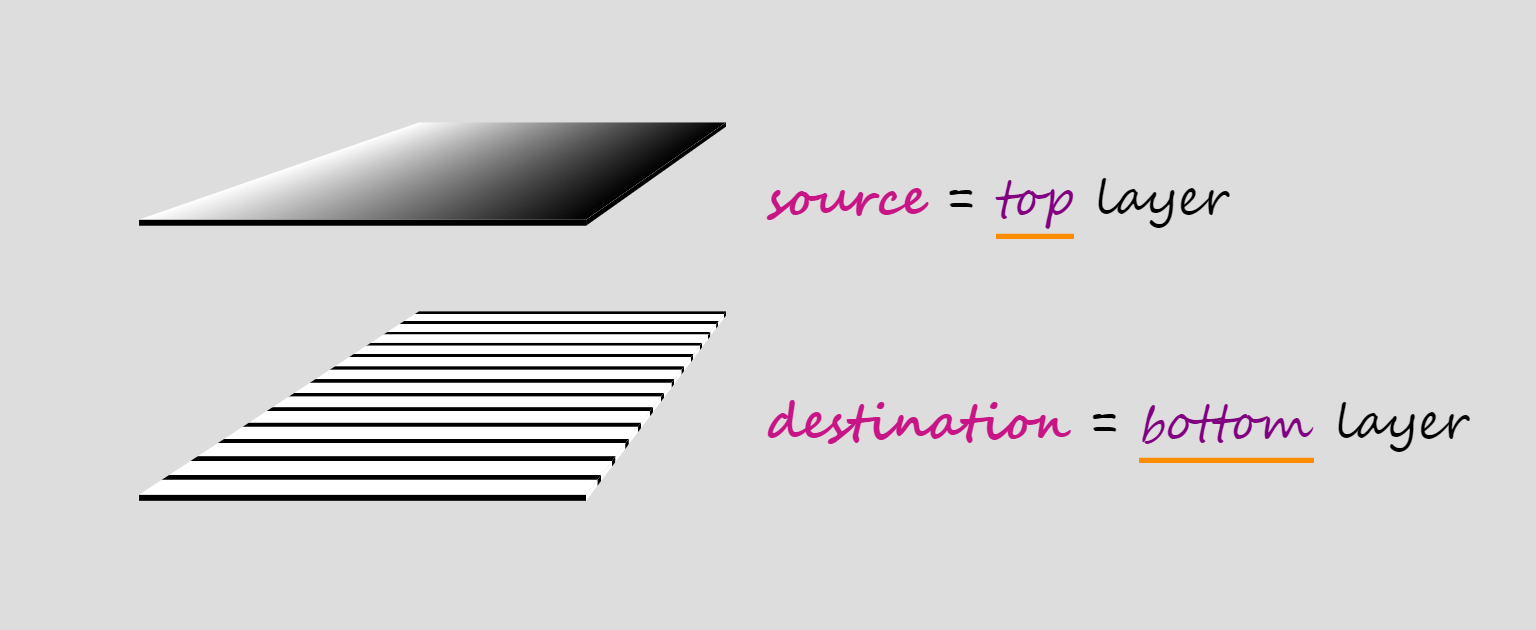Compositing terminology.

When we have more than two layers, compositing is done in stages, starting from the bottom.

In a first stage, the second layer from the bottom is our source and the first layer from the bottom is our destination. These two layers get composited and the result becomes the destination for the second stage, where the third layer from the bottom is the source. Compositing the third layer with the result of compositing the first two gives us the destination for the third stage, where the fourth layer from the bottom is the source.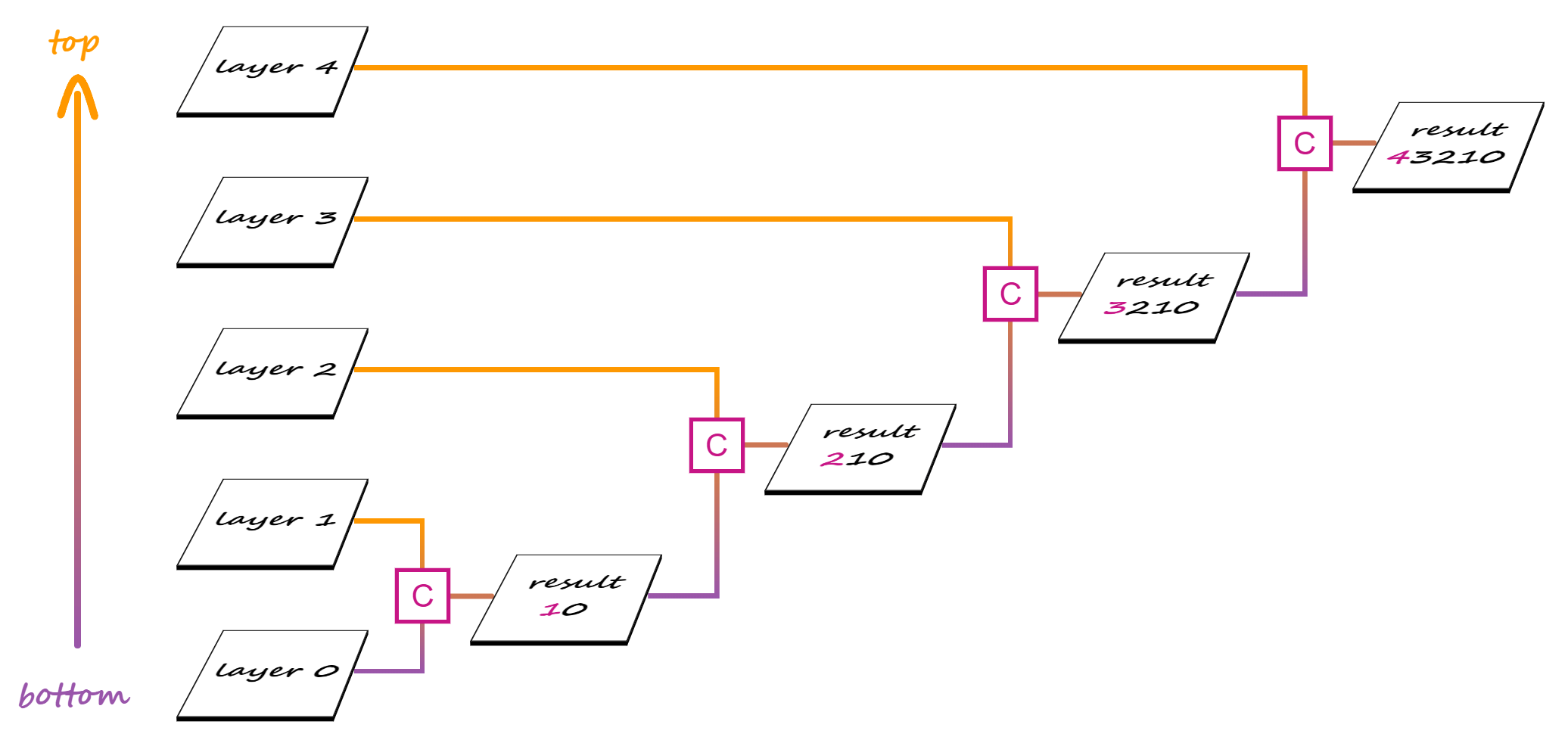Compositing multiple layers.

And so on, until we get to the final stage, where the topmost layer is composited with the result of compositing all the layers beneath.

### Why mask compositing is useful

Both CSS and SVG masks have their limitations, their advantages and disadvantages. We can go around the limitations of SVG masks by using CSS masks, but, due to CSS masks working differently from SVG masks, taking the CSS route leaves us unable to achieve certain results without compositing.

In order to better understand all of this, let’s consider the following image of a pawesome Siberian tiger cub:The image we want to have masked on our page.

And let’s say we want to get the following masking effect on it: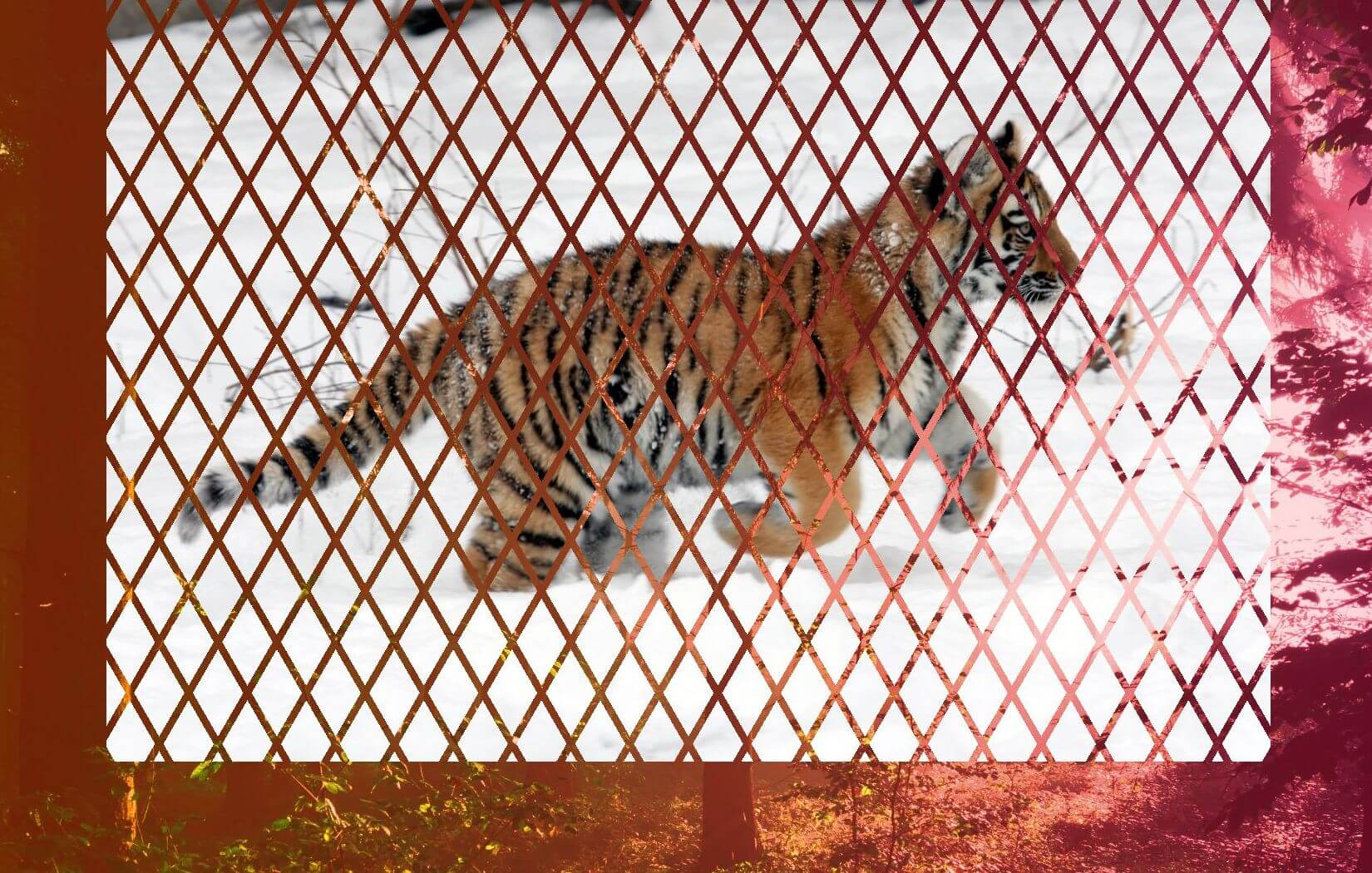Desired result.

This particular `mask` keeps the rhombic shapes visible, while the lines separating them get masked and we can see through the image to the element behind.

We also want this masking effect to be flexible. We don’t want to be tied to the image’s dimensions or aspect ratio and we want to be able to easily switch (just by changing a `%` value to a `px` one) in between a `mask` that scales with the image and one that doesn’t.

In order to do this, we first need to understand how SVG and CSS masks each work and what we can and cannot do with them.

#### SVG masking

SVG masks are `luminance` masks by default. This means that the pixels of the masked element corresponding to the `white` `mask` pixels are fully opaque, the pixels of the masked element corresponding to `black` `mask` pixels are fully `transparent` and the pixels of the masked element corresponding to `mask` pixels somewhere in between `black` and `white` in terms of luminance (`grey`, `pink`, `lime`) are semitransparent.

The formula used to get the luminance out of a given RGB value is:
`.2126·R + .7152·G + .0722·B`

For our particular example, this means we need to make the rhombic areas `white` and the lines separating them `black`, creating the pattern that can be seen below: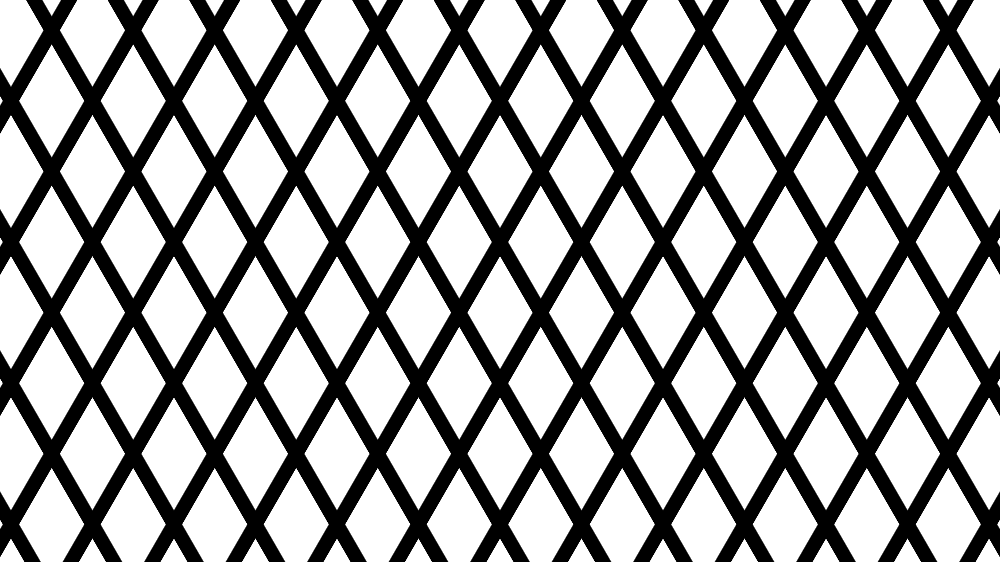Black and white rhombic pattern used as an SVG mask.

In order to get the pattern above, we start with a `white` SVG rectangle element `rect`. Then, one might think we need to draw lots of black lines… but we don’t! Instead, we only add a `path` made up of the two diagonals of this rectangle and ensure its `stroke` is `black`.

To create the first diagonal (top left to bottom right), we use a “move to” (`M`) command to the top left corner, followed by a “line to” (`L`) command to the bottom right corner.

To create the second diagonal (top right to bottom left), we use a “move to” (`M`) command to the top right corner, followed by a “line to” (`L`) command to the bottom left corner.

Our code so far is:

``````svg(viewBox=[0, 0, w, h].join(' '))
rect(width=w height=h fill='#fff')
path(d=`M0 0
L\${w} \${h}

M\${w} 0
L0 \${h}` stroke='#000')``````

The result so far doesn’t seem to look anything like the rhombic pattern we want to get…

See the Pen by thebabydino (@thebabydino) on CodePen.

… but that’s about to change! We increase the thickness (`stroke-width`) of the `black` diagonal lines and make them dashed with the gaps between the dashes (`7%`) bigger than the dashes themselves (`1%`).

``````svg(viewBox=[0, 0, w, h].join(' '))
rect(width=w height=h fill='#fff')
path(d=`M0 0
L\${w} \${h}

M\${w} 0
L0 \${h}` stroke='#000'
stroke-width='15%'
stroke-dasharray='1% 7%')``````

Can you now see where this is going?

See the Pen by thebabydino (@thebabydino) on CodePen.

If we keep increasing the thickness (`stroke-width`) of our `black` diagonal lines to a value like `150%`, then they end up covering the entire rectangle and giving us the pattern we’ve been after!

See the Pen by thebabydino (@thebabydino) on CodePen.

Now we can wrap our `rect` and `path` elements inside a `mask` element and apply this `mask` on whatever element we wish – in our case, the tiger image.

``````svg(viewBox=[0, 0, w, h].join(' '))
mask#m
rect(width=w height=h fill='#fff')
path(d=`M0 0
L\${w} \${h}

M\${w} 0
L0 \${h}` stroke='#000'
stroke-width='15%'
stroke-dasharray='1% 7%')
img(src='image.jpg' width=w)``````
``img { mask: url(#m) }``

The above should work. But sadly, things are not perfect in practice. At this point, we only get the expected result in Firefox (live demo). Even worse, not getting the desired masked pattern in Chrome doesn’t mean our element stays as it is unmasked – applying this `mask` makes it disappear altogether! Of course, since Chrome needs the `-webkit-` prefix for the `mask` property (when used on HTML elements), not adding the prefix means that it doesn’t even try to apply the `mask` on our element.

The most straightforward workaround for `img` elements is to turn them into SVG `image` elements.

``````svg(viewBox=[0, 0, w, h].join(' ') width=w)
mask#m
rect(width=w height=h fill='#fff')
path(d=`M0 0
L\${w} \${h}

M\${w} 0
L0 \${h}` stroke='#000'
stroke-width='15%'
stroke-dasharray='1% 7%')
image(xlink:href=url width=w mask='url(#m)')``````

See the Pen by thebabydino (@thebabydino) on CodePen.

This gives us the result we’ve been after, but if we want to `mask` another HTML element, not an `img` one, things get a bit more complicated as we’d need to include it inside the SVG with `foreignObject`.

Even worse, with this solution, we’re hardcoding dimensions and this always feels yucky.

Of course, we can make the `mask` ridiculously large so that it’s unlikely there may be an image it couldn’t cover. But that feels just as bad as hardcoding dimensions.

We can also try tackling the hardcoding issue by switching the `maskContentUnits` to `objectBoundingBox`:

``````svg(viewBox=[0, 0, w, h].join(' '))
mask#m(maskContentUnits='objectBoundingBox')
rect(width=1 height=1 fill='#fff')
path(d=`M0 0
L1 1

M1 0
L0 1` stroke='#000'
stroke-width=1.5
stroke-dasharray='.01 .07')
image(xlink:href=url width='100%' mask='url(#m)')``````

But we’re still hardcoding the dimensions in the `viewBox` and, while their actual values don’t really matter, their aspect ratio does. Furthermore, our masking pattern is now created within a `1x1` square and then stretched to cover the masked element.

Shape stretching means shape distortion, which is why is why our rhombic shapes don’t look as they did before anymore.

See the Pen by thebabydino (@thebabydino) on CodePen.

Ugh.

We can tweak the start and end points of the two lines making up our path:

``````svg(viewBox=[0, 0, w, h].join(' '))
mask#m
rect(width=1 height=1 fill='#fff')
path(d=`M-.75 0
L1.75 1

M1.75 0
L-.75 1` stroke='#000'
stroke-width=1.5
stroke-dasharray='.01 .07')
image(xlink:href=url width='100%' mask='url(#m)')``````

See the Pen by thebabydino (@thebabydino) on CodePen.

However, in order to get one particular rhombic pattern, with certain angles for our rhombic shapes, we need to know the image’s aspect ratio.

Sigh. Let’s just drop it and see what we can do with CSS.

#### CSS masking

CSS masks are `alpha` masks by default. This means that the pixels of the masked element corresponding to the fully opaque `mask` pixels are fully opaque, the pixels of the masked element corresponding to the fully `transparent` `mask` pixels are fully `transparent` and the pixels of the masked element corresponding to semitransparent `mask` pixels are semitransparent. Basically, each and every pixel of the masked element gets the alpha channel of the corresponding `mask` pixel.

For our particular case, this means making the rhombic areas opaque and the lines separating them `transparent`, so let’s how can we do that with CSS gradients!

In order to get the pattern with `white` rhombic areas and `black` separating lines, we can layer two repeating linear gradients:

See the Pen by thebabydino (@thebabydino) on CodePen.

``````repeating-linear-gradient(-60deg,
#000 0, #000 5px, transparent 0, transparent 35px),
repeating-linear-gradient(60deg,
#000 0, #000 5px, #fff 0, #fff 35px)``````

This is the pattern that does the job if we have a `luminance` `mask`.

But in the case of an `alpha` `mask`, it’s not the `black` pixels that give us full transparency, but the `transparent` ones. And it’s not the `white` pixels that give us full opacity, but the fully opaque ones – `red`, `black`, `white`… they all do the job! I personally tend to use `red` or `tan` as this means only three letters to type and the fewer letters to type, the fewer opportunities for awful typos that can take half an hour to debug.

So the first idea is to apply the same technique to get opaque rhombic areas and `transparent` separating lines. But in doing so, we run into a problem: the opaque parts of the second gradient layer cover parts of the first layer we’d like to still keep `transparent` and the other way around.

See the Pen by thebabydino (@thebabydino) on CodePen.

So what we’re getting is pretty far from opaque rhombic areas and transparent separating lines.

My initial idea was to use the pattern with `white` rhombic areas and `black` separating lines, combined with setting `mask-mode` to `luminance` to solve the problem by making the CSS mask work like an SVG one.

This property is only supported by Firefox, though there is the non-standard `mask-source-type` for WebKit browsers. And sadly, support is not even the biggest issue as neither the standard Firefox way, nor the non-standard WebKit way give us the result we’re after (live demo).

Fortunately, `mask-composite` is here to help! So let’s see what values this property can take and what effect they each have.

### `mask-composite` values and what they do

First, we decide upon two gradient layers for our `mask` and the image we want masked.

The two gradient `mask` layers we use to illustrate how each value of this property works are as follows:

``````--l0: repeating-linear-gradient(90deg,
red, red 1em,
transparent 0, transparent 4em);
--l1: linear-gradient(red, transparent);

mask: var(--l1) /* top (source) layer */,
var(--l0) /* bottom (destination) layer */``````

These two layers can be seen as `background` gradients in the Pen below (note that the `body` has a hashed `background` so that the `transparent` and semitransparent gradient areas are more obvious):

See the Pen by thebabydino (@thebabydino) on CodePen.

The layer on top (`--l1`) is the source, while the bottom layer (`--l0`) is the destination.

We apply the `mask` on this image of a gorgeous Amur leopard.The image we apply the mask on.

Alright, now that we got that out of the way, let’s see what effect each `mask-composite` value has!

#### `add`

This is the `initial` value, which gives us the same effect as not specifying `mask-composite` at all. What happens in this case is that the gradients are added one on top of the other and the resulting `mask` is applied.

Note that, in the case of semitransparent `mask` layers, the alphas are not simply added, in spite of the value name. Instead, the following formula is used, where `α₁` is the alpha of the pixel in the source (top) layer and `α₀` is the alpha of the corresponding pixel in the destination (bottom) layer:

``α₁ + α₀ – α₁·α₀``

Wherever at least one `mask` layer is fully opaque (its alpha is `1`), the resulting `mask` is fully opaque and the corresponding pixels of the masked element are shown fully opaque (with an alpha of `1`).

If the source (top) layer is fully opaque, then `α₁` is `1`, and replacing in the formula above, we have:

``1 + α₀ - 1·α₀ = 1 + α₀ - α₀ = 1``

If the destination (bottom) layer is fully opaque, then `α₀` is `1`, and we have:

``α₁ + 1 – α₁·1 = α₁ + 1 – α₁ = 1``

Wherever both `mask` layers are fully `transparent` (their alphas are `0`), the resulting `mask` is fully `transparent` and the corresponding pixels of the masked element are therefore fully `transparent` (with an alpha of `0`) as well.

``0 + 0 – 0·0 = 0 + 0 + 0 = 0``

Below, we can see what this means for the `mask` layers we’re using – what the layer we get as a result of compositing looks like and the final result that applying it on our Amur leopard image produces.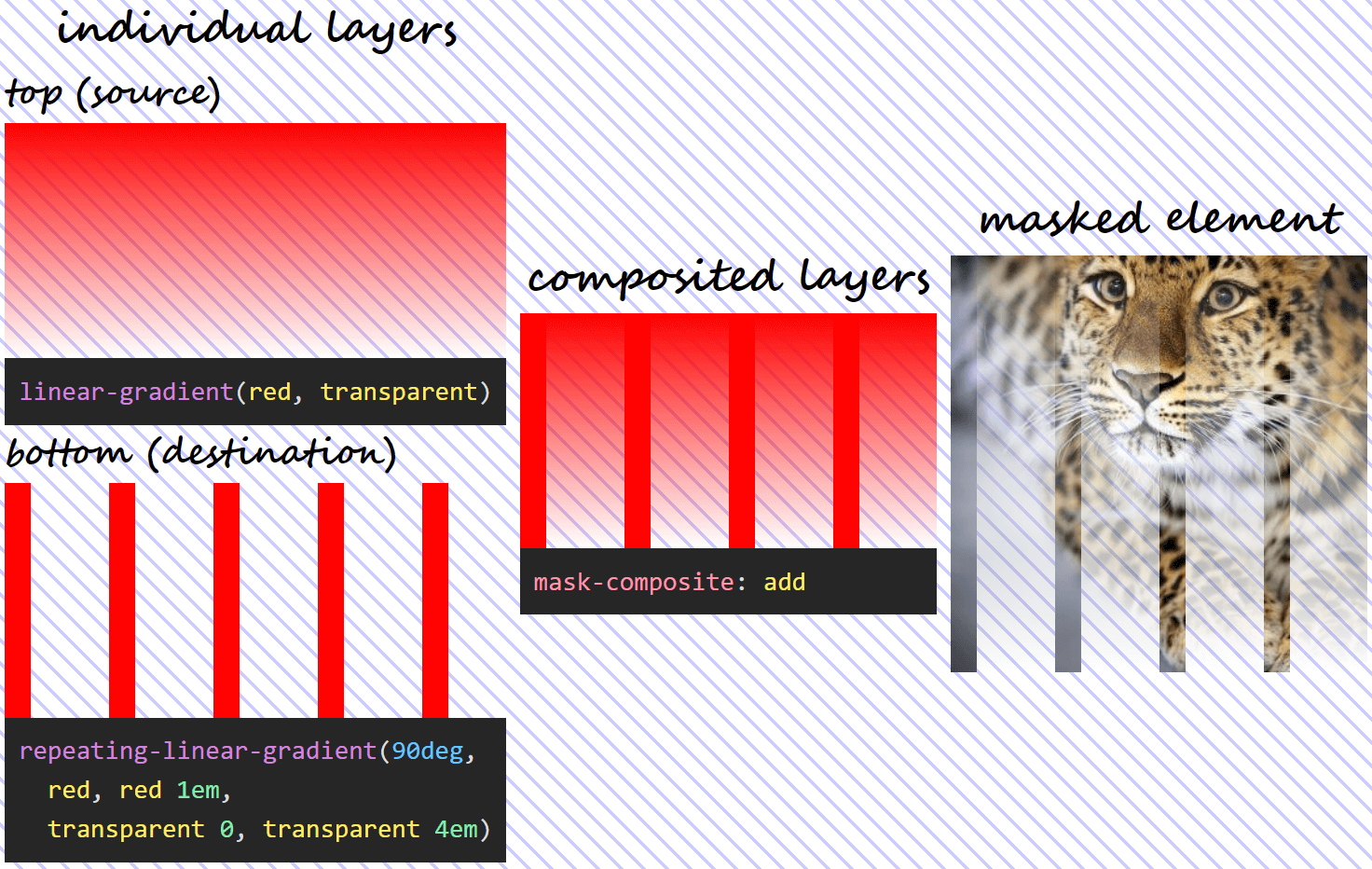What using mask-composite: add for two given layers does.

#### `subtract`

The name refers to “subtracting” the destination (layer below) out of the source (layer above). Again, this does not refer to simply capped subtraction, but uses the following formula:

``α₁·(1 – α₀)``

The above formula means that, since anything multiplied with `0` gives us `0`, wherever the source (top) layer is fully `transparent` or wherever the destination (bottom) layer is fully opaque, the resulting `mask` is also fully `transparent` and the corresponding pixels of the masked element are also fully `transparent`.

If the source (top) layer is fully `transparent`, replacing its alpha with `0` in our formula gives us:

``0·(1 – α₀) = 0``

If the destination (bottom) layer is fully opaque, replacing its alpha with `1` in our formula gives us:

``α₁·(1 – 1) = α₁·0 = 0``

This means using the previously defined `mask` and setting `mask-composite: subtract`, we get the following:What using mask-composite: subtract for two given layers does.

Note that, in this case, the formula isn’t symmetrical, so, unless `α₁` and `α₀` are equal, we don’t get the same thing if we swap the two mask layers (`α₁·(1 – α₀)` isn’t the same as `α₀·(1 – α₁)`). This means we have a different visual result if we swap the order of the two layers!Using mask-composite: subtract when the two given layers have been swapped.

#### `intersect`

In this case, we only see the pixels of the masked element from where the two `mask` layers intersect. The formula used is the product between the alphas of the two layers:

``α₁·α₀``

What results from the formula above is that, wherever either `mask` layer is fully `transparent` (its alpha is `0`), the resulting `mask` is also fully `transparent` and so are the corresponding pixels of the masked element.

If the source (top) layer is fully `transparent`, replacing its alpha with `0` in our formula gives us:

``0·α₀ = 0``

If the destination (bottom) layer is fully `transparent`, replacing its alpha with `0` in our formula gives us:

``α₁·0 = 0``

Also, wherever both `mask` layers are fully opaque (their alphas are `1`), the resulting `mask` is fully opaque and so are the corresponding pixels of the masked element. This because, if the alphas of the two layers are both `1`, we have:

``1·1 = 1``

In the particular case of our `mask`, setting `mask-composite: intersect` means we have:What using mask-composite: intersect for two given layers does.

#### `exclude`

In this case, each layer is basically excluded from the other, with the formula being:

``α₁·(1 – α₀) + α₀·(1 – α₁)``

In practice, this formula means that, wherever both `mask` layers are fully `transparent` (their alphas are `0`) or fully opaque (their alphas are `1`), the resulting `mask` is fully `transparent` and the corresponding pixels of the masked element are fully `transparent` as well.

If both `mask` layers are fully `transparent`, our replacing their alphas with `0` in our formula results in:

``0·(1 – 0) + 0·(1 – 0) = 0·1 + 0·1 = 0 + 0 = 0``

If both `mask` layers are fully opaque, our replacing their alphas with `1` in our formula results in:

``1·(1 – 1) + 1·(1 – 1) = 1·0 + 1·0 = 0 + 0 = 0``

It also means that, wherever one layer is fully `transparent` (its alpha is `0`), while the other one is fully opaque (its alpha is `1`), then the resulting `mask` is fully opaque and so are the corresponding pixels of the masked element.

If the source (top) layer is fully `transparent`, while the destination (bottom) layer is fully opaque, replacing `α₁` with `0` and `α₀` with `1` gives us:

``0·(1 – 1) + 1·(1 – 0) = 0·0 + 1·1 = 0 + 1 = 1``

If the source (top) layer is fully opaque, while the destination (bottom) layer is fully `transparent`, replacing `α₁` with `1` and `α₀` with `0` gives us:

``1·(1 – 0) + 0·(1 – 1) = 1·1 + 0·0 = 1 + 0 = 1``

With our `mask`, setting `mask-composite: exclude` means we have:What using mask-composite: exclude for two given layers does.

### Applying this to our use case

We go back to the two gradients we attempted to get the rhombic pattern with:

``````--l1: repeating-linear-gradient(-60deg,
transparent 0, transparent 5px,
tan 0, tan 35px);
--l0: repeating-linear-gradient(60deg,
transparent 0, transparent 5px,
tan 0, tan 35px)``````

If we make the completely opaque (`tan` in this case) parts semitransparent (let’s say `rgba(tan, .5)`), the visual result gives us an indication of how compositing could help here:

``````\$c: rgba(tan, .5);
\$sw: 5px;

--l1: repeating-linear-gradient(-60deg,
transparent 0, transparent #{\$sw},
#{\$c} 0, #{\$c} #{7*\$sw});
--l0: repeating-linear-gradient(60deg,
transparent 0, transparent #{\$sw},
#{\$c} 0, #{\$c} #{7*\$sw})``````

See the Pen by thebabydino (@thebabydino) on CodePen.

The rhombic areas we’re after are formed at the intersection between the semitransparent strips. This means using `mask-composite: intersect` should do the trick!

``````\$sw: 5px;

--l1: repeating-linear-gradient(-60deg,
transparent 0, transparent #{\$sw},
tan 0, tan #{7*\$sw});
--l0: repeating-linear-gradient(60deg,
transparent 0, transparent #{\$sw},
tan 0, tan #{7*\$sw});
mask: var(--l1) intersect, var(--l0)``````

Note that we can even include the compositing operation in the shorthand! Which is something I really love, because the fewer chances of wasting at least ten minutes not understanding why `masj-composite`, `msdk-composite`, `nask-composite`, `mask-comoisite` and the likes don’t work, the better!

Not only does this give us the desired result, but, if now that we’ve stored the `transparent` strip width into a variable, changing this value to a `%` value (let’s say `\$sw: .05%`) makes the mask scale with the image!

If the `transparent` strip width is a `px` value, then both the rhombic shapes and the separating lines stay the same size as the image scales up and down with the viewport.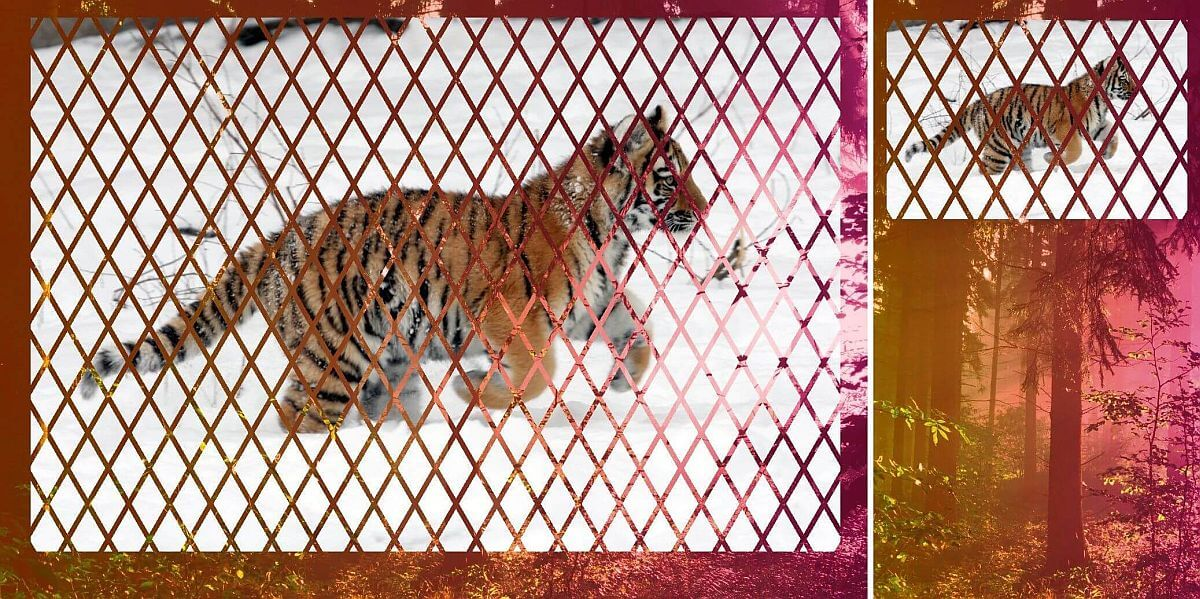Masked image at two different viewport widths when the transparent separating lines in between the rhombic shapes have a px-valued width.

If the `transparent` strip width is a `%` value, then both the rhombic shapes and the separating lines are relative in size to the image and therefore scale up and down with it.Masked image at two different viewport widths when the transparent separating lines in between the rhombic shapes have a %-valued width.

Too good to be true? What’s the support for this?

The bad news is that `mask-composite` is only supported by Firefox at the moment. The good news is we have an alternative for WebKit browsers, so we can extend the support.

### Extending support

WebKit browsers support (and have supported for a long, long time) a non-standard version of this property, `-webkit-mask-composite` which needs different values to work. These equivalent values are:

• `source-over` for `add`
• `source-out` for `subtract`
• `source-in` for `intersect`
• `xor` for `exclude`

So, in order to have a cross-browser version, all we need to is add the WebKit version as well, right?

Well, sadly, things are not that simple.

First off, we cannot use this value in the `-webkit-mask` shorthand, the following does not work:

``-webkit-mask: var(--l1) source-in, var(--l0)``

And if we take the compositing operation out of the shorthand and write the longhand after it, as seen below:

``````-webkit-mask: var(--l1), var(--l0);
-webkit-mask-composite: source-in;
mask: var(--l1) intersect, var(--l0)``````

… the entire image completely disappears!

And if you think that’s weird, check this: using any of the other three operations `add`/ `source-over`, `subtract`/ `source-out`, `exclude`/ `xor`, we get the expected result in WebKit browsers as well as in Firefox. It’s only the `source-in` value that breaks things in WebKit browsers!

See the Pen by thebabydino (@thebabydino) on CodePen.

What gives?!

Why is this particular value breaking things in WebKit?

When I first came across this, I spent a few good minutes trying to find a typo in `source-in`, then copy pasted it from a reference, then from a second one in case the first reference got it wrong, then from a third… and then I finally had another idea!

It appears as if, in the case of the non-standard WebKit alternative, we also have compositing applied between the layer at the bottom and a layer of nothing (considered completely `transparent`) below it.

For the other three operations, this makes absolutely no difference. Indeed, adding, subtracting or excluding nothing doesn’t change anything. If we are to take the formulas for these three operations and replace `α₀` with `0`, we always get `α₁`:

• `add`/ `source-over`: `α₁ + 0 – α₁·0 = α₁ + 0 - 0 = α₁`
• `subtract`/ `source-out`: `α₁·(1 – 0) = α₁·1 = α₁`
• `exclude`/ `xor`: `α₁·(1 – 0) + 0·(1 – α₁) = α₁·1 + 0 = α₁`

However, intersection with nothing is a different story. Intersection with nothing is nothing! This is something that’s also illustrated by replacing `α₀` with `0` in the formula for the `intersect`/ `source-in` operation:

``α₁·0 = 0``

The alpha of the resulting layer is `0` in this case, so no wonder our image gets completely masked out!

So the first fix that came to mind was to use another operation (doesn’t really matter which of the other three, I picked `xor` because it has fewer letters and it can be fully selected by double clicking) for compositing the layer at the bottom with this layer of nothing below it:

``````-webkit-mask: var(--l1), var(--l0);
-webkit-mask-composite: source-in, xor;
mask: var(--l1) intersect, var(--l0)``````

And yes, this does work!

You can resize the embed below to see how the `mask` behaves when it scales with the image and when it doesn’t.

See the Pen by thebabydino (@thebabydino) on CodePen.

Note that we need to add the non-standard WebKit version before the standard one so that when WebKit browsers finally implement the standard version as well, this overrides the non-standard one.

Well, that’s about it! I hope you’ve enjoyed this article and learned something new from it.

### A couple more demos

Before closing, here are two more demos showcasing why `mask-composite` is cool.

The first demo shows a bunch of 1 element umbrellas. Each “bite” is created with a `radial-gradient()` that we `exclude` from the full circular shape. Chrome has a little rendering issue, but the result looks perfect in Firefox.1 element umbrellas using mask-composite (live demo).

The second demo shows three 1 element loaders (though only the second two use `mask-composite`). Note that the `animation` only works in Chrome here as it needs Houdini.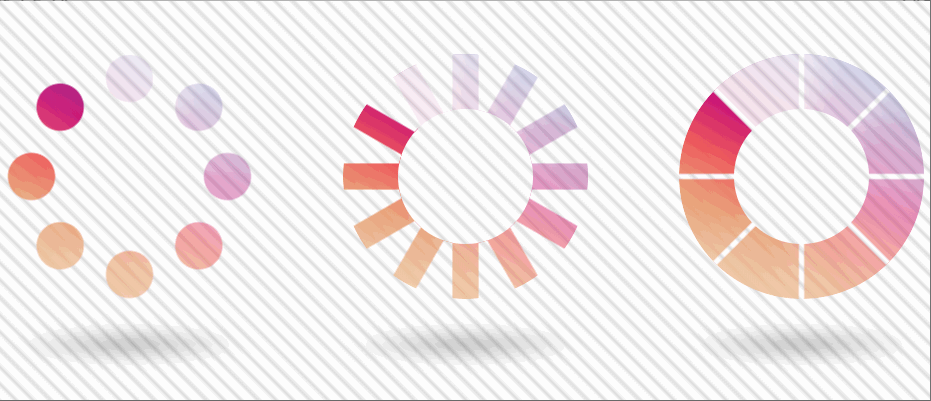1 element loaders using mask-composite (live demo).

How about you – what other use cases can you think of?